# 吴恩达机器学习作业Python实现(一)：线性回归

## 单变量线性回归

%matplotlib inline

import numpy as np
import pandas as pd
import matplotlib.pyplot as plt


path =  'ex1data1.txt'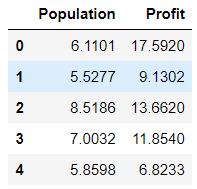data.describe()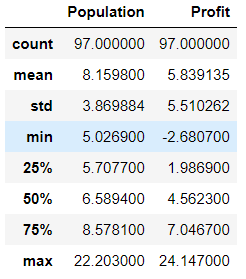(你在现实生活中遇到的许多其他问题都是多维度的，不能在二维图上画出来。)

data.plot(kind='scatter', x='Population', y='Profit', figsize=(8,5))
plt.show()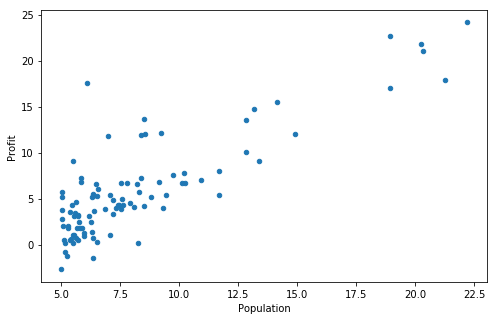$J\left( \theta \right)=\frac{1}{2m}\sum\limits_{i=1}^{m}{{{\left( {{h}_{\theta }}\left( {{x}^{(i)}} \right)-{{y}^{(i)}} \right)}^{2}}}$

def computeCost(X, y, theta):
inner = np.power(((X * theta.T) -  y), 2)
return np.sum(inner) / (2 * len(X))


data.insert(0, 'Ones', 1)


# set X (training data) and y (target variable)
cols = data.shape  # 列数
X = data.iloc[:,0:cols-1]  # 取前cols-1列，即输入向量
y = data.iloc[:,cols-1:cols] # 取最后一列，即目标向量


X.head()  # head()是观察前5行

y.head()


array的优势就是不仅仅表示二维，还能表示3、4、5…维，而且在大部分Python程序里，array也是更常用的。

1. 对应元素相乘：matrix可以用np.multiply(X2,X1)，array直接X1*X2
2. 点乘：matrix直接X1*X2，array可以 X1@X2 或 X1.dot(X2) 或 np.dot(X1, X2)

X = np.matrix(X.values)
y = np.matrix(y.values)
theta = np.matrix([0,0])


theta 是一个(1,2)矩阵

np.array([[0,0]]).shape
# (1, 2)


X.shape, theta.shape, y.shape
# ((97, 2), (1, 2), (97, 1))


computeCost(X, y, theta) # 32.072733877455676


$J\left( \theta \right)=\frac{1}{2m}\sum\limits{i=1}^{m}{{{\left( {{h}{\theta }}\left( {{x}^{(i)}} \right)-{{y}^{(i)}} \right)}^{2}}}$

${{h}_{\theta }}\left( x \right)={{\theta }^{T}}X={{\theta }_{0}}{{x}_{0}}+{{\theta }_{1}}{{x}_{1}}+{{\theta }_{2}}{{x}_{2}}+...+{{\theta }_{n}}{{x}_{n}}$

${{\theta }_{j}}:={{\theta }_{j}}-\alpha \frac{\partial }{\partial {{\theta }_{j}}}J\left( \theta \right)$

$\theta_j:=\theta_j-\alpha\frac{1}{m}\sum^{m}_{i=1}(h_\theta(x^{(i)}) - y^{(i)})x_j^{(i)}$

X.shape, theta.shape, y.shape, X.shape
# ((97, 2), (1, 2), (97, 1), 97)

def gradientDescent(X, y, theta, alpha, epoch):
"""reuturn theta, cost"""

temp = np.matrix(np.zeros(theta.shape))  # 初始化一个 θ 临时矩阵(1, 2)
parameters = int(theta.flatten().shape)  # 参数 θ的数量
cost = np.zeros(epoch)  # 初始化一个ndarray，包含每次epoch的cost
m = X.shape  # 样本数量m

for i in range(epoch):
# 利用向量化一步求解
temp =theta - (alpha / m) * (X * theta.T - y).T * X

# 以下是不用Vectorization求解梯度下降
#         error = (X * theta.T) - y  # (97, 1)

#         for j in range(parameters):
#             term = np.multiply(error, X[:,j])  # (97, 1)
#             temp[0,j] = theta[0,j] - ((alpha / m) * np.sum(term))  # (1,1)

theta = temp
cost[i] = computeCost(X, y, theta)

return theta, cost


alpha = 0.01
epoch = 1000


final_theta, cost = gradientDescent(X, y, theta, alpha, epoch)


computeCost(X, y, final_theta)


np.linspace()在指定的间隔内返回均匀间隔的数字。

x = np.linspace(data.Population.min(), data.Population.max(), 100)  # 横坐标
f = final_theta[0, 0] + (final_theta[0, 1] * x)  # 纵坐标，利润

fig, ax = plt.subplots(figsize=(6,4))
ax.plot(x, f, 'r', label='Prediction')
ax.scatter(data['Population'], data.Profit, label='Traning Data')
ax.legend(loc=2)  # 2表示在左上角
ax.set_xlabel('Population')
ax.set_ylabel('Profit')
ax.set_title('Predicted Profit vs. Population Size')
plt.show()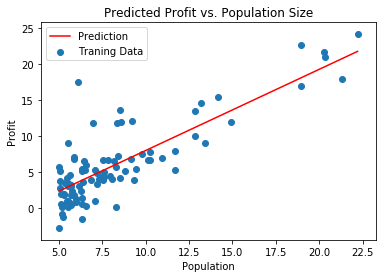fig, ax = plt.subplots(figsize=(8,4))
ax.plot(np.arange(epoch), cost, 'r')  # np.arange()返回等差数组
ax.set_xlabel('Iterations')
ax.set_ylabel('Cost')
ax.set_title('Error vs. Training Epoch')
plt.show()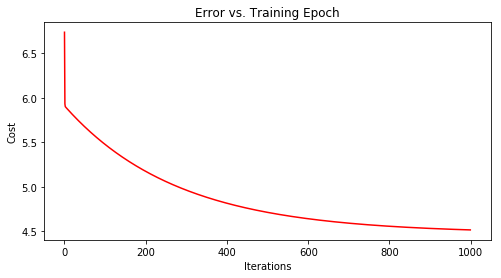## 多变量线性回归

path =  'ex1data2.txt'
data2 = pd.read_csv(path, names=['Size', 'Bedrooms', 'Price'])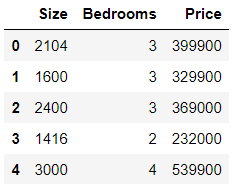data2 = (data2 - data2.mean()) / data2.std()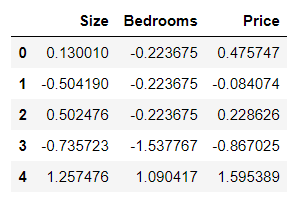# add ones column
data2.insert(0, 'Ones', 1)

# set X (training data) and y (target variable)
cols = data2.shape
X2 = data2.iloc[:,0:cols-1]
y2 = data2.iloc[:,cols-1:cols]

# convert to matrices and initialize theta
X2 = np.matrix(X2.values)
y2 = np.matrix(y2.values)
theta2 = np.matrix(np.array([0,0,0]))

# perform linear regression on the data set
g2, cost2 = gradientDescent(X2, y2, theta2, alpha, epoch)

# get the cost (error) of the model
computeCost(X2, y2, g2), g2


fig, ax = plt.subplots(figsize=(12,8))
ax.plot(np.arange(epoch), cost2, 'r')
ax.set_xlabel('Iterations')
ax.set_ylabel('Cost')
ax.set_title('Error vs. Training Epoch')
plt.show()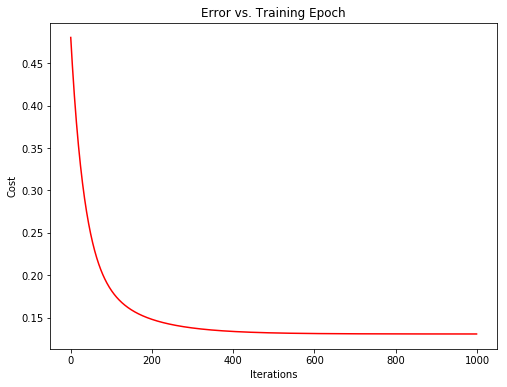from sklearn import linear_model
model = linear_model.LinearRegression()
model.fit(X, y)


scikit-learn model的预测表现

x = np.array(X[:, 1].A1)
f = model.predict(X).flatten()

fig, ax = plt.subplots(figsize=(8,5))
ax.plot(x, f, 'r', label='Prediction')
ax.scatter(data.Population, data.Profit, label='Traning Data')
ax.legend(loc=2)
ax.set_xlabel('Population')
ax.set_ylabel('Profit')
ax.set_title('Predicted Profit vs. Population Size')
plt.show()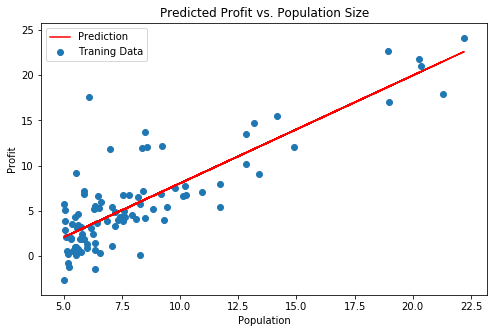## normal equation（正规方程）

# 正规方程
def normalEqn(X, y):
theta = np.linalg.inv(X.T@X)@X.T@y#X.T@X等价于X.T.dot(X)
return theta

final_theta2=normalEqn(X, y)#感觉和批量梯度下降的theta的值有点差距
final_theta2

#梯度下降得到的结果是matrix([[-3.24140214,  1.1272942 ]])


#### 机器学习必学的10大算法

08-1412-052万+

#### 吴恩达机器学习课后作业深度解析（附答案）（ex1）

11-291915

#### 吴恩达机器学习第一次作业（python实现）：线性回归

10-252万+

#### 机器学习：吴恩达Coursera机器学习课后作业答案MATLAB和Python版本分享

05-252173

#### 吴恩达机器学习 线性回归 作业(房价预测) Python实现 代码详细解释

03-21563

#### 吴恩达机器学习总结：第一课 单变量线性回归（大纲摘要及课后作业）

09-163985

#### 吴恩达机器学习课程作业——ML_ex1

12-091万+

#### 吴恩达机器学习笔记（一），含作业及附加题答案链接

04-19679

#### 吴恩达老师机器学习第一次作业：线性回归【python实现】

10-233万+

#### 吴恩达机器学习与深度学习作业目录 [图片已修复]©️2020 CSDN 皮肤主题: 编程工作室 设计师: CSDN官方博客点击重新获取扫码支付1.余额是钱包充值的虚拟货币，按照1:1的比例进行支付金额的抵扣。
2.余额无法直接购买下载，可以购买VIP、C币套餐、付费专栏及课程。余额充值